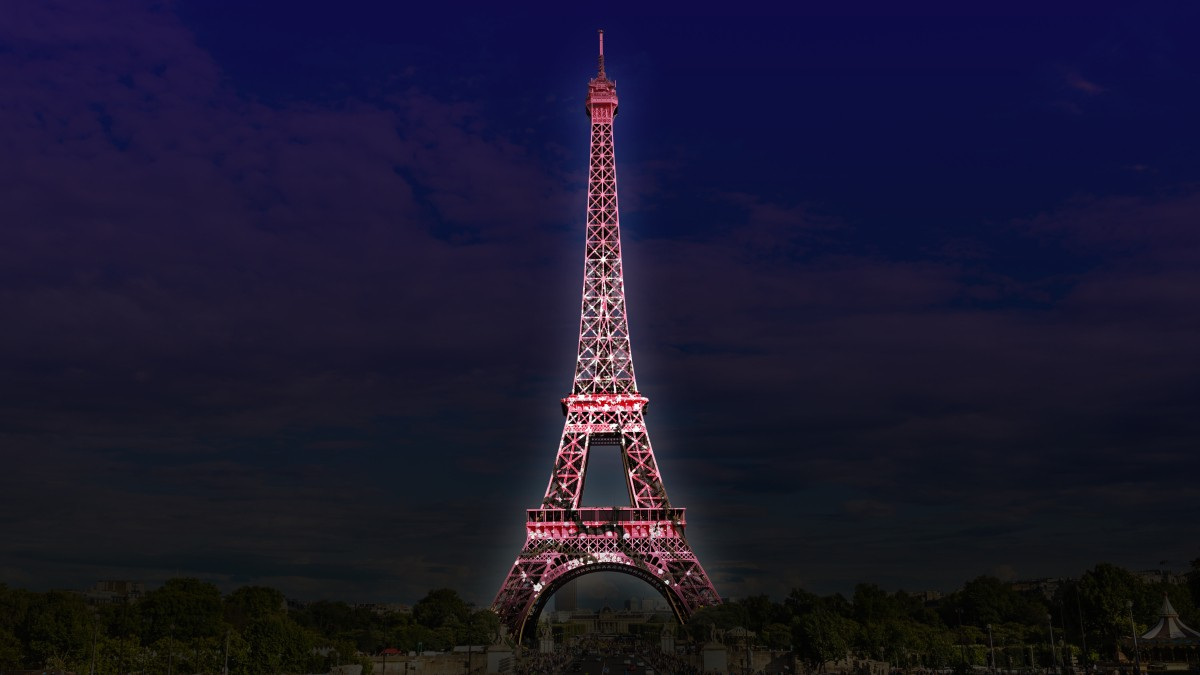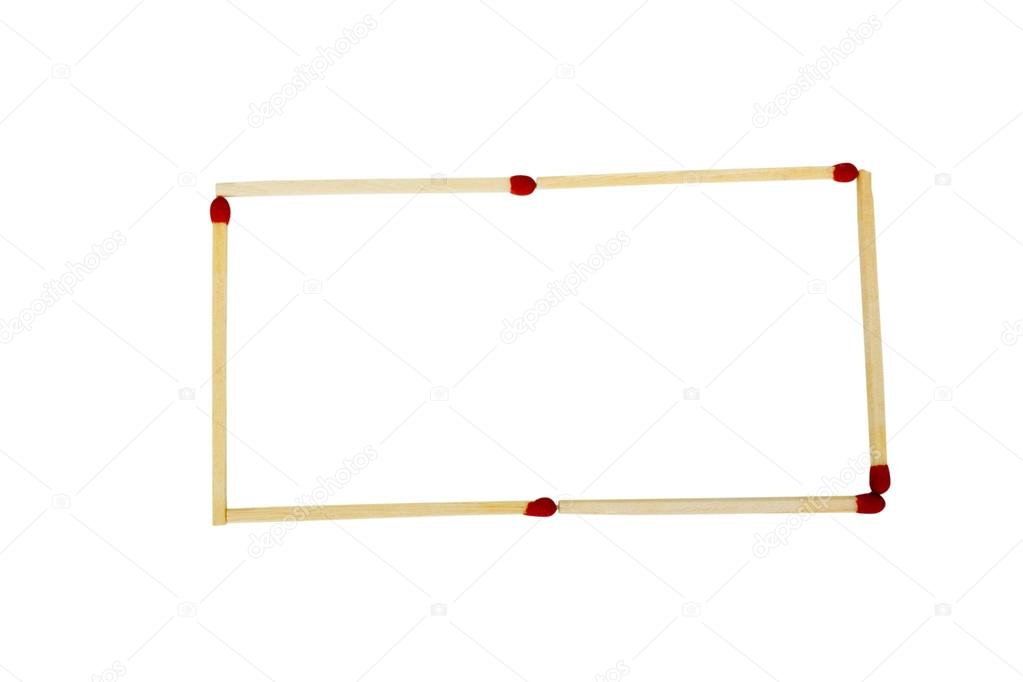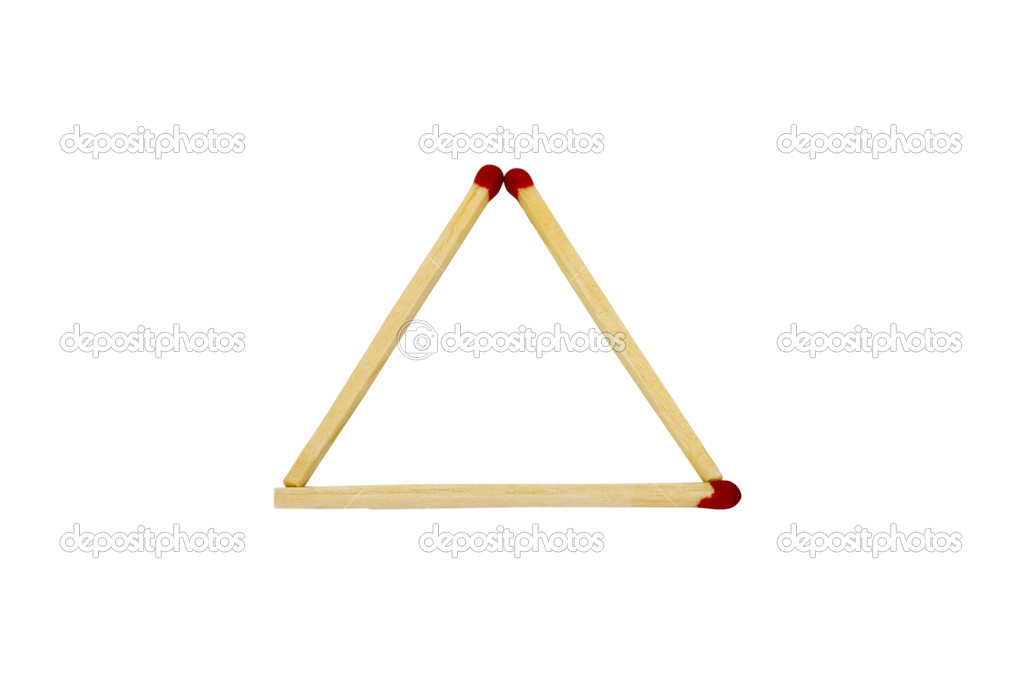We see the Eiffel tower all the basic shapes are a triangle and also pylons near our house are also contain a number of triangles why they choose triangle for their construction? because the triangle is the strongest geometry in nature unlike squares and rectangle they have a constant angle and they equally spread the applied force by all the three directions. You think the circle is stronger yes is it but it useful in a tank of compressed air, water. In the case of the triangle they useful in the beam-like structure. Triangle used for strengthening the structure, especially in the square.

The strongest angle for the structure is 45 degree the diagonal of the square have to angle by adding two triangles. For these qualities, architects use these kinds of shape in their structure ex: Egyptian pyramids.

### ANALYZE YOUR SELF:

Make a triangle, square and rectangular shapes by using firesticks and cellophane tape or glue. Now apply the same force on the one side of each object and calculate the time taken by the objects to break. From this, we can determine the strongest structure for the architecture.